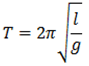# On what factors does the time period of a simple pendulum depend?

The time period of a simple pendulum is given bySo, time period of simple pendulum depends upon the length of the pendulum, acceleration due to gravity and the temperature (as length depends on temperature). It is directly proportional to the square root of length and inversely proportional to the square root of acceleration due to gravity.